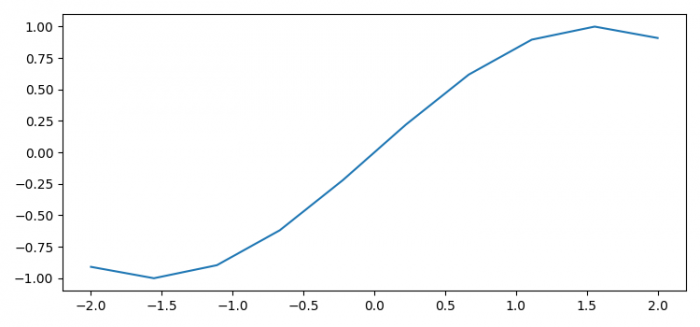# How to turn off the upper/right axis tick marks in Matplotlib?

To turn off the upper or right axis ticks marks in matplotlib, we can make a custom dictionary visible_ticks and turn off the flag.

## Steps

• Set the figure size and adjust the padding between and around the subplots.
• Create x and y data points using numpy.
• Plot x and y data points using plot() method.
• Make a dictionary to turn off the axis ticks marks.
• To display the figure, use show() method.

## Example

import numpy as np
import matplotlib.pyplot as plt

plt.rcParams["figure.figsize"] = [7.50, 3.50]
plt.rcParams["figure.autolayout"] = True
x = np.linspace(-2, 2, 10)
y = np.sin(x)
plt.plot(x, y)
visible_ticks = {
"top": False,
"right": False
}
plt.tick_params(axis="x", which="both", **visible_ticks)
plt.show()

## Output